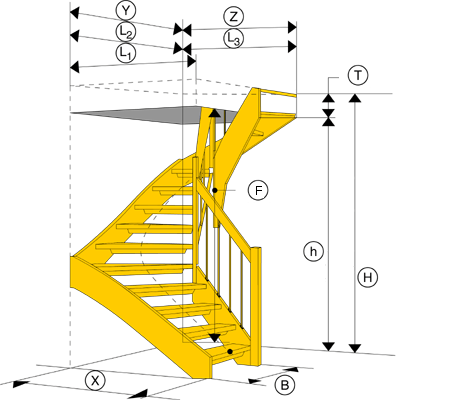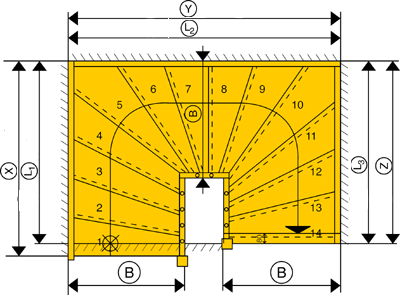# Required space and important measures for a half-turned staircase.

How much space does a Half-turned staircase require?

Description:
A convenient staircase with the going 230 mm calculated in the middle of the staircase.
For wider stairs, the tread line is moved inwards and takes up more space
The measurements shown below include the end tread of 80 mm but not the 30 mm nosing of the string at the start.

How to calculate:

1. Measure your total height from floor below to floor upstairs (H)
2. Choose the number of rises. (For example, if you have a floor height of 2650 mm, you choose 14 step heights.)
3. Choose the width of the staircase. (B).
4. The measurement you will find in the table is the total sum of the horizontal lengths according to the drawing (X) and (Y). The flexibility is that you can adjust any horizontal length as long as the total sum is the same as the value in the table.How to measure a half-turned staircase:
Designations

H = Stair height – measured vertically from the floor below to the floor upstairs
h = Room height – measured from the floor below to the
T = Well thickness.

(with the measurments of the room height and the well thickness you can calculate the head room)

B = The total staircase width. .
X = The horizontal measurement of the first flight of the staircase.
Y = The horizontal measurement of the second flight of the staircase.
Z = The horizontal measurement of the third flight of the staircase.

L1 = The horizontal measurement of the well opening at the X-measurement of the staircase.
L2 = The horizontal measurement of the well opening at the Y-measurement of the staircase.
L3 = The horizontal measurement of the well opening at the Z-measurement of the staircase.

(It doesn’t matter if you have plenty of space for the stairs downstairs.
The size of the stairwell – and the height of the room – determines how large the stairs you can have.)